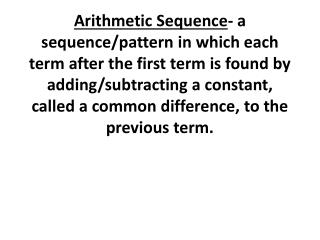Download PresentationArithmetic Sequence Formula: a n = a 1 + ( n – 1)d

Arithmetic Sequence Formula: a n = a 1 + ( n – 1)d - PowerPoint PPT Presentation

Arithmetic Sequence - a sequence/pattern in which each term after the first term is found by adding/subtracting a constant, called a common difference, to the previous term. Arithmetic Sequence Formula: a n = a 1 + ( n – 1)d.I am the owner, or an agent authorized to act on behalf of the owner, of the copyrighted work described.
Download PresentationArithmetic Sequence Formula: a n = a 1 + ( n – 1)d

Download Policy: Content on the Website is provided to you AS IS for your information and personal use and may not be sold / licensed / shared on other websites without getting consent from its author.While downloading, if for some reason you are not able to download a presentation, the publisher may have deleted the file from their server.

- - - - - - - - - - - - - - - - - - - - - - - - - - E N D - - - - - - - - - - - - - - - - - - - - - - - - - -
Presentation Transcript
1. Arithmetic Sequence- a sequence/pattern in which each term after the first term is found by adding/subtracting a constant, called a common difference, to the previous term.

2. Arithmetic Sequence Formula: an = a1 + ( n – 1)d an = the term that you are looking for or the last term in the sequence. a1 = the first term in the sequence. d = the value that is added to each term to get the next term. n = the nth term in the sequence. (always positive.) For example, what is the 4th term.

3. Determine whether each sequence is arithmetic. If so, identify the common difference. • 1.1, 4, 7, 10, … • 2. 1, 3, 6, 10, 15, … 3. − 16, − 19, − 22, − 25, -28, …

4. Find the 24th term of each arithmetic sequence. • 1. 4, 6, 8, 10, 12, … 2. 9, 5, 1, − 3, − 7, … • an = a1 + ( n – 1)d

5. Find the position of the given term in each arithmetic sequence 1. Which term is -324 in the sequence, 5, -2, -9, -16, -23, …. 2. 187 is the nth term in the sequence, 7, 13, 19, 25, ……

6. An architect is designing a building with sides in the shape of a trapezoid. The number of windows on each floor formsan arithmetic sequence. There are 124 windows on the first floor and 116 windows on the second floor. a. Write an explicit formula to represent the sequence. b. How many windows are on the tenth floor?